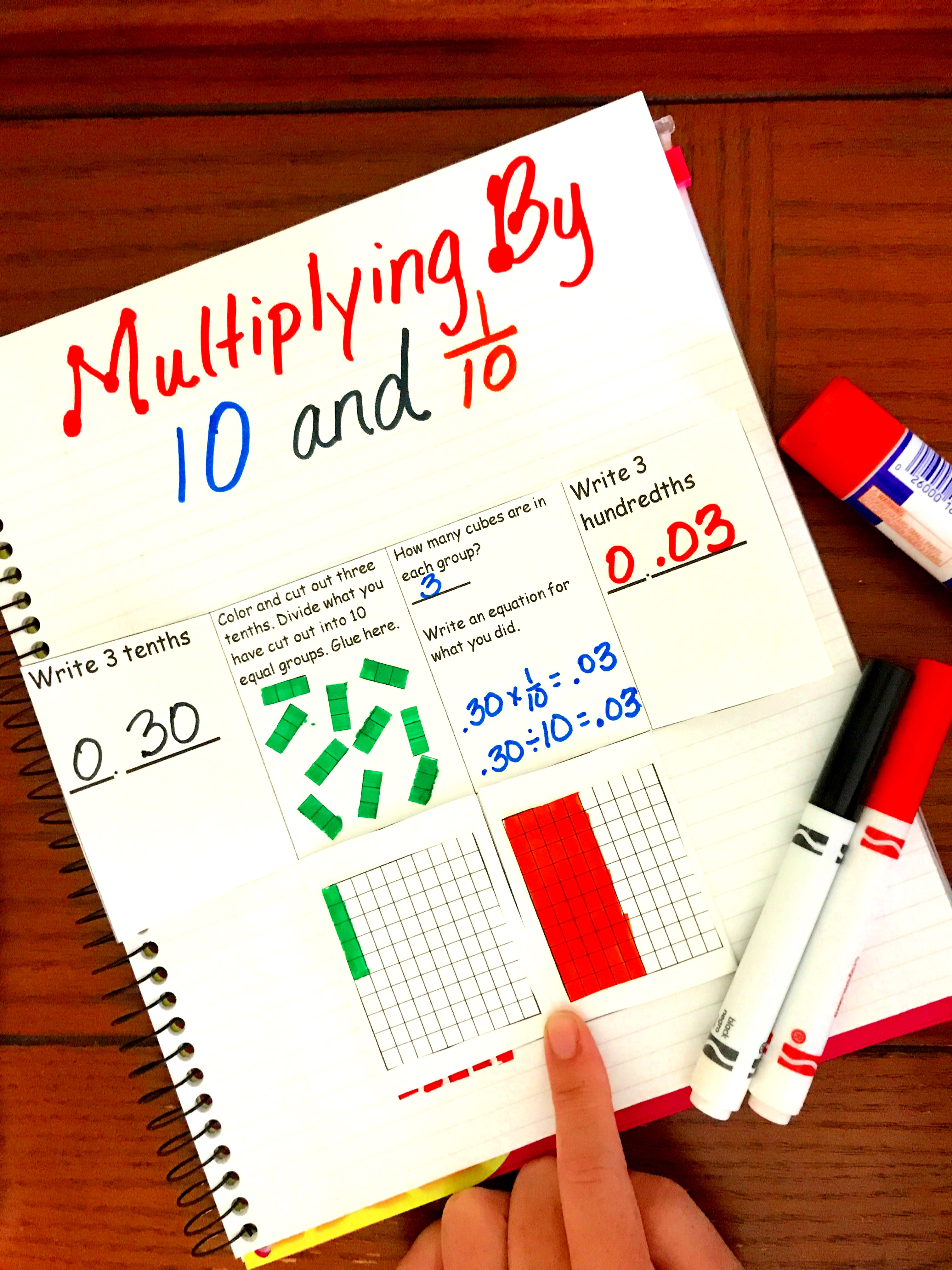# Multiplying Decimals | Multiplying 10 vs .10 | Free Printable | Interactive Notebook

## Multiplying Decimals

This interactive notebook will help you teach multiplying decimals by finding digit values with decimals and what happens when you multiply a digit by 10 vs .10. Grab this free printable today.

The class is silent. Nothing is heard except for the teacher’s voice droning on like a scene from Charlie Brown. “The value of digits changes as they move up or down in a number.

A digit in one place represents 10 times as much as it represents in the place to its right and 1/10 of what it represents in the place to its left.”

Students are doodling in their notebooks, twirling their hair, and ordering the newest game off of Amazon Prime.

*This post contains Amazon affiliated links

## Finding Digit Value with Decimals

The lesson continues with a few examples drawn on the board, and then the dreaded, “Take out your math books and practice how digits change in a number.”

The students dutifully pull out their books, but moans fill the air. The page rustle and the chatter of numerous children fill the classroom as they get ready to answer the 20 questions on the page.

Years ago, this is how many math classes may have looked. You might argue that it worked as the children were doing the work, and even getting the questions right.

## How to Multiply with a Decimal: Issues Students Face

The big question is, do they understand the concept?

Some children who are gifted with a mathematical brain will instantly see the connection. The majority of students will be able to answer the questions, but not have a clue why or even what they are doing.

Our little ones that struggle may not even be able to do the problem without some hands-on instruction.

I don’t want my students to lack an understanding of any math concept. I definitely don’t want my precious students that I spend numerous hours a week with, to walk away from my classroom not understanding the why.

If you are taking the time to read this, I know you don’t want that either.

## Manipulatives for Decimals

At the moment, I’m teaching the cutest Kindergarten student (yes, I’m a little biased), but the majority of my teaching career has been spent in upper elementary.

As upper-grade teachers, we often get into the mindset that manipulatives and place value are for those early years, but they are just as important in 3-5 as they were in k-2.

Much of fourth and fifth grade is spent with fractions and decimals, so it is imperative that our students are seeing how the place value they learned in 2nd grade corresponds with decimals.

One way is when our kiddos work on finding the value of a decimal. We can do this by teaching what happens when a number is moved from one place to the left or to the right, in other words, what happens when a number is multiplied by 10 vs .10.

So what happens when a digit in the one’s place is moved one place to the right? You guessed it, it is multiplied by 10.

What happens when you move a digit from one place to the left? Yep, you have divided by 10!

## How to Teach Multiplying Decimals

So how do you teach this? Get out those base ten blocks and play with them. Get out grid paper and have the children color and cut to help them understand what is happening when Multiplying 10 vs .10

1. If you are using grids, grab some scissors and coloring pencils.
2. Start off with any number like eight-tenths, and have the students color in that number.
3. Next, ask them to draw 10 circles in their notebook and divide the eight-tenths up equally into the circles.
4. At this point comes one of the hardest things for me to do. Step away, and see what they can do on their own. If a student is totally stuck, you can ask how many cubes are in each row. This may give them an idea, but if they continue to be totally at a loss, suggest they cut out every cube and place one in each circle.

Now ask them what number is represented in each circle. In a perfect world, you should get the answer eight-hundredths. The value of the digit eight changed as it moved to the hundredths place.

Now, instead of telling your students that a digit is 1/10 of what it represents in the place to its left, you have shown them and allowed them to explore it.

## How to Multiply a Decimal by .10

You can do the same teaching the opposite way, and this way is even easier.

1.  Start by having each child color in five hundredths, and explain that they will be multiplying five-hundredths by 10.
2. Since your students are multiplying by 10 have them draw 10 circles and place five hundredths in each circle.
3. Finally, your students will figure out how many cubes they now have in total.

## Multiplying Interactive Notebook

I’ve provided a free interactive notebook to help you teach multiplying 10 vs .10.

• Simply begin by printing off the free printable
• Next cut the printable squares and glue the four middle squares into an interactive notebook
• Next cut on the line in between the two squares
• Have them color in 3/10 and 3/100 on two different flats. Glue the 3/10 flat on the top left tab and the 3/100 on the top right tab.
• Have your students open up the tabs and follow the instructions.

## Worksheet on Multiplication of Decimals

See below for the free multiplying decimals worksheets. You can use these worksheets to create a multiplication interactive notebook. If you need more decimal practice check out my Decimal Multiplication With Arrays and Lattice Work.

Multiplying-10-vs-.10